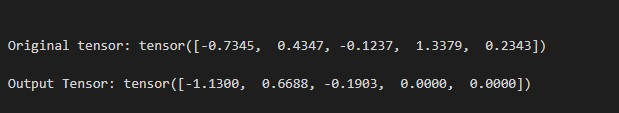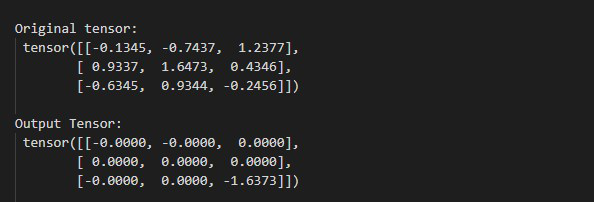GeeksforGeeks App
Open AppBrowser
Continue

# How to Use torch.nn.Dropout() Method in Python PyTorch

In this article, we are going to discuss how you use torch.nn.Dropout() Method in Python PyTorch.

## torch.nn.Dropout() Method

In PyTorch, torch.nn.Dropout() method randomly replaced some of the elements of an input tensor by 0 with a given probability. This method only supports the non-complex-valued inputs. before moving further let’s see the syntax of the given method.

Syntax: torch.nn.Dropout(p=0.5, inplace=False)

Parameters:

• P: P is the probability of an element is replaced with 0 or not. Default value of P is 0.5
• inplace: This will used to do this operation in-place. Default value of inplace is False.

Return: This method return a tensor after replaced some of the elements of input tensor by 0 with a given probability P.

Example 1:

In this example, we will use torch.nn.Dropout() method with probability 0.35. It means there is a 35% chance of an element of input tensor to be replaced with 0.

## Python

 `# Import required library``import` `torch` `# define a tensor``tens ``=` `torch.tensor([``-``0.7345``, ``0.4347``, ``-``0.1237``,``                     ``1.3379``, ``0.2343``])` `# print the tensor``print``(``"Original tensor:"``, tens)` `# use torch.nn.Dropout() method with``# probability p=0.35``drop ``=` `torch.nn.Dropout(.``35``)``Output_tens ``=` `drop(tens)` `# Display Output``print``(``" Output Tensor:"``, Output_tens)`

Output:Example 2:

In this example, we will use torch.nn.Dropout() method with probability is 0.85 and in place is True. It means there is an 85% chance of an element of input tensor to be replaced with 0.

## Python

 `# Import the required library``import` `torch` `# define a tensor``tens ``=` `torch.tensor([[``-``0.1345``, ``-``0.7437``, ``1.2377``],``                     ``[``0.9337``, ``1.6473``, ``0.4346``],``                     ``[``-``0.6345``, ``0.9344``, ``-``0.2456``]])` `# print the tensor``print``(``"\n Original tensor: \n"``, tens)` `# use torch.nn.Dropout() method with``# probability p=0.85``# perform this operation in-place by``# using inplace=True``drop ``=` `torch.nn.Dropout(.``85``)``Output_tens ``=` `drop(tens)` `# Display Tensor``print``(``"\n Output Tensor: \n"``, Output_tens)`

Output:My Personal Notes arrow_drop_up